Browse by Topic
Related Topics

# Computational Knowledge and the Future of Pure Mathematics

Every four years for more than a century there’s been an International Congress of Mathematicians (ICM) held somewhere in the world. In 1900 it was where David Hilbert announced his famous collection of math problems—and it’s remained the top single periodic gathering for the world’s research mathematicians.

This year the ICM is in Seoul, and I’m going to it today. I went to the ICM once before—in Kyoto in 1990. Mathematica was only two years old then, and mathematicians were just getting used to it. Plenty already used it extensively—but at the ICM there were also quite a few who said, “I do pure mathematics. How can Mathematica possibly help me?”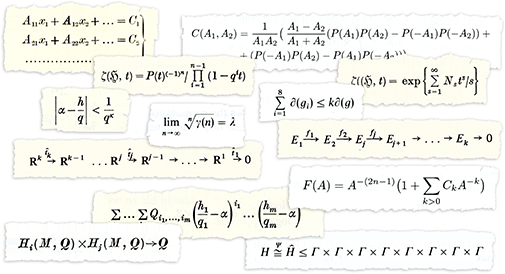Twenty-four years later, the vast majority of the world’s pure mathematicians do in fact use Mathematica in one way or another. But there’s nevertheless a substantial core of pure mathematics that still gets done pretty much the same way it’s been done for centuries—by hand, on paper.

Ever since the 1990 ICM I’ve been wondering how one could successfully inject technology into this. And I’m excited to say that I think I’ve recently begun to figure it out. There are plenty of details that I don’t yet know. And to make what I’m imagining real will require the support and involvement of a substantial set of the world’s pure mathematicians. But if it’s done, I think the results will be spectacular—and will surely change the face of pure mathematics at least as much as Mathematica (and for a younger generation, Wolfram|Alpha) have changed the face of calculational mathematics, and potentially usher in a new golden age for pure mathematics.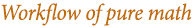The whole story is quite complicated. But for me one important starting point is the difference in the typical workflows for calculational mathematics and pure mathematics. Calculational mathematics tends to involve setting up calculational questions, and then working through them to get results—just like in typical interactive Mathematica sessions. But pure mathematics tends to involve taking mathematical objects, results or structures, coming up with statements about them, and then giving proofs to show why those statements are true.

How can we usefully insert technology into this workflow? Here’s one simple way. Think about Wolfram|Alpha. If you enter 2+2, Wolfram|Alpha—like Mathematica—will compute 4. But if you enter new york—or, for that matter, 2.3363636 or cos(x) log(x)—there’s no single “answer” for it to compute. And instead what it does is to generate a report that gives you a whole sequence of “interesting facts” about what you entered.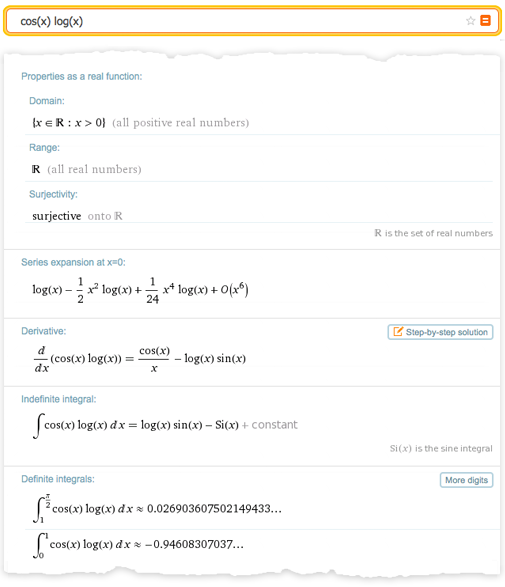And this kind of thing fits right into the workflow for pure mathematics. You enter some mathematical object, result or structure, and then the system tries to tell you interesting things about it—just like some extremely wise mathematical colleague might. You can guide the system if you want to, by telling it what kinds of things you want to know about, or even by giving it a candidate statement that might be true. But the workflow is always the Wolfram|Alpha-like “what can you tell me about that?” rather than the Mathematica-like “what’s the answer to that?”

Wolfram|Alpha already does quite a lot of this kind of thing with mathematical objects. Enter a number, or a mathematical expression, or a graph, or a probability distribution, or whatever, and Wolfram|Alpha will use often-quite-sophisticated methods to try to tell you a collection of interesting things about it.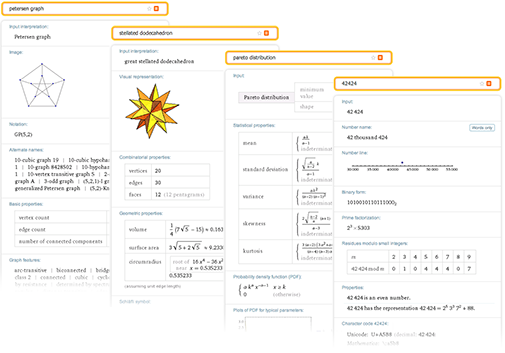But to really be useful in pure mathematics, there’s something else that’s needed. In addition to being able to deal with concrete mathematical objects, one also has to be able to deal with abstract mathematical structures.

Countless pure mathematical papers start with things like, “Let F be a field with such-and-such properties.” We need to be able to enter something like this—then have our system automatically give us interesting facts and theorems about F, in effect creating a whole automatically generated paper that tells the story of F.

So what would be involved in creating a system to do this? Is it even possible? There are several different components, all quite difficult and time consuming to build. But based on my experiences with Mathematica, Wolfram|Alpha, and A New Kind of Science, I am quite certain that with the right leadership and enough effort, all of them can in fact be built.

A key part is to have a precise symbolic description of mathematical concepts and constructs. Lots of this now already exists—after more than a quarter century of work—in Mathematica. Because built right into the Wolfram Language are very general ways to represent geometries, or equations, or stochastic processes or quantifiers. But what’s not built in are representations of pure mathematical concepts like bijections or abstract semigroups or pullbacks.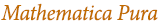Over the years, plenty of mathematicians have implemented specific cases. But could we systematically extend the Wolfram Language to cover the whole range of pure mathematics—and make a kind of “Mathematica Pura”? The answer is unquestionably yes. It’ll be fascinating to do, but it’ll take lots of difficult language design.

I’ve been doing language design now for 35 years—and it’s the hardest intellectual activity I know. It requires a curious mixture of clear thinking, aesthetics and pragmatic judgement. And it involves always seeking the deepest possible understanding, and trying to do the broadest unification—to come up in the end with the cleanest and “most obvious” primitives to represent things.

Today the main way pure mathematics is described—say in papers—is through a mixture of mathematical notation and natural language, together with a few diagrams. And in designing a precise symbolic language for pure mathematics, this has to be the starting point.

One might think that somehow mathematical notation would already have solved the whole problem. But there’s actually only a quite small set of constructs and concepts that can be represented with any degree of standardization in mathematical notation—and indeed many of these are already in the Wolfram Language.

So how should one go further? The first step is to understand what the appropriate primitives are. The whole Wolfram Language today has about 5000 built-in functions—together with many millions of built-in standardized entities. My guess is that to broadly support pure mathematics there would need to be something less than a thousand other well-designed functions that in effect define frameworks—together with maybe a few tens of thousands of new entities or their analogs.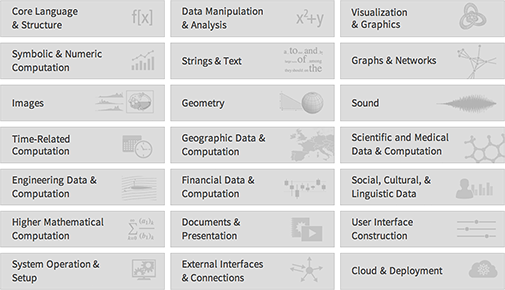Take something like function spaces. Maybe there’ll be a FunctionSpace function to represent a function space. Then there’ll be various operations on function spaces, like PushForward or MetrizableQ. Then there’ll be lots of named function spaces, like “CInfinity”, with various kinds of parameterizations.

Underneath, everything’s just a symbolic expression. But in the Wolfram Language there end up being three immediate ways to input things, all of which are critical to having a convenient and readable language. The first is to use short notations—like + or —as in standard mathematical notation. The second is to use carefully chosen function names—like MatrixRank or Simplex. And the third is to use free-form natural language—like trefoil knot or aleph0.

One wants to have short notations for some of the most common structural or connective elements. But one needs the right number: not too few, like in LISP, nor too many, like in APL. Then one wants to have function names made of ordinary words, arranged so that if one’s given something written in the language one can effectively just “read the words” to know at least roughly what’s going on in it.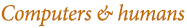But in the modern Wolfram Language world there’s also free-form natural language. And the crucial point is that by using this, one can leverage all the various convenient—but sloppy—notations that actual mathematicians use and find familiar. In the right context, one can enter “L2” for Lebesgue Square Integrable—and the natural language system will take care of disambiguating it and inserting the canonical symbolic underlying form.

Ultimately every named construct or concept in pure mathematics needs to have a place in our symbolic language. Most of the 13,000+ entries in MathWorld. Material from the 5600 or so entries in the MSC2010 classification scheme. All the many things that mathematicians in any given field would readily recognize when told their names.

But, OK, so let’s say we manage to create a precise symbolic language that captures the concepts and constructs of pure mathematics. What can we do with it?

One thing is to use it “Wolfram|Alpha style”: you give free-form input, which is then interpreted into the language, and then computations are done, and a report is generated.

But there’s something else too. If we have a sufficiently well-designed symbolic language, it’ll be useful not only to computers but also to humans. In fact, if it’s good enough, people should prefer to write out their math in this language than in their current standard mixture of natural language and mathematical notation.

When I write programs in the Wolfram Language, I pretty much think directly in the language. I’m not coming up with a description in English of what I’m trying to do and then translating it into the Wolfram Language. I’m forming my thoughts from the beginning in the Wolfram Language—and making use of its structure to help me define those thoughts.

If we can develop a sufficiently good symbolic language for pure mathematics, then it’ll provide something for pure mathematicians to think in too. And the great thing is that if you can describe what you’re thinking in a precise symbolic language, there’s never any ambiguity about what anything means: there’s a precise definition that you can just go to the documentation for the language to find.

And once pure math is represented in a precise symbolic language, it becomes in effect something on which computation can be done. Proofs can be generated or checked. Searches for theorems can be done. Connections can automatically be made. Chains of prerequisites can automatically be found.

But, OK, so let’s say we have the raw computational substrate we need for pure mathematics. How can we use this to actually implement a Wolfram|Alpha-like workflow where we enter descriptions of things, and then in effect automatically get mathematical wisdom about them?

There are two seemingly different directions one can go. The first is to imagine abstractly enumerating possible theorems about what has been entered, and then using heuristics to decide which of them are interesting. The second is to start from computable versions of the millions of theorems that have actually been published in the literature of mathematics, and then figure out how to connect these to whatever has been entered.

Each of these directions in effect reflects a slightly different view of what doing mathematics is about. And there’s quite a bit to say about each direction.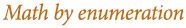Let’s start with theorem enumeration. In the simplest case, one can imagine starting from an axiom system and then just enumerating true theorems based on that system. There are two basic ways to do this. The first is to enumerate possible statements, and then to use (implicit or explicit) theorem-proving technology to try to determine which of them are true. And the second is to enumerate possible proofs, in effect treeing out possible ways the axioms can be applied to get theorems.

It’s easy to do either of these things for something like Boolean algebra. And the result is that one gets a sequence of true theorems. But if a human looks at them, many of them seem trivial or uninteresting. So then the question is how to know which of the possible theorems should actually be considered “interesting enough” to be included in a report that’s generated.

My first assumption was that there would be no automatic approach to this—and that “interestingness” would inevitably depend on the historical development of the relevant area of mathematics. But when I was working on A New Kind of Science, I did a simple experiment for the case of Boolean algebra.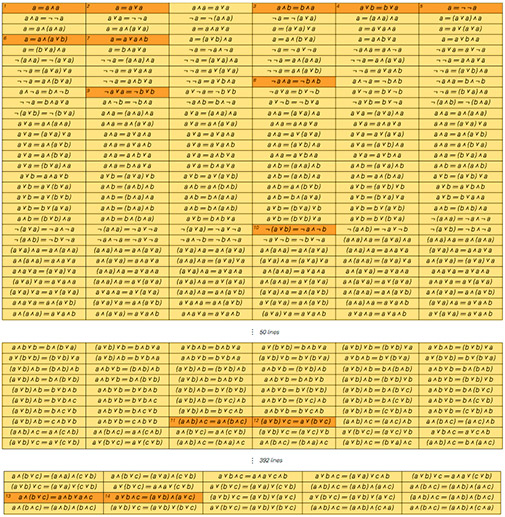There are 14 theorems of Boolean algebra that are usually considered “interesting enough” to be given names in textbooks. I took all possible theorems and listed them in order of complexity (number of variables, number of operators, etc). And the surprising thing I found is that the set of named theorems corresponds almost exactly to the set of theorems that can’t be proved just from ones that precede them in the list. In other words, the theorems which have been given names are in a sense exactly the minimal statements of new information about Boolean algebra.

Boolean algebra is of course a very simple case. And in the kind of enumeration I just described, once one’s got the theorems corresponding to all the axioms, one would conclude that there aren’t any more “interesting theorems” to find—which for many mathematical theories would be quite silly. But I think this example is a good indication of how one can start to use automated heuristics to figure out which theorems are “worth reporting on”, and which are, for example, just “uninteresting embellishments”.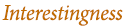Of course, the general problem of ranking “what’s interesting” comes up all over Wolfram|Alpha. In mathematical examples, one’s asking what region is interesting to plot?, “what alternate forms are interesting?” and so on. When one enters a single number, one’s also asking “what closed forms are interesting enough to show?”—and to know this, one for example has to invent rankings for all sorts of mathematical objects (how complicated should one consider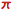relative to log(343) relative to Khinchin’s Constant, and so on?).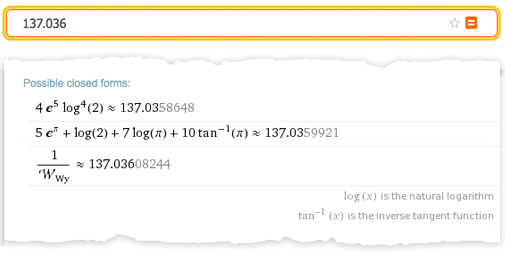So in principle one can imagine having a system that takes input and generates “interesting” theorems about it. Notice that while in a standard Mathematica-like calculational workflow, one would be taking input and “computing an answer” from it, here one’s just “finding interesting things to say about it”.

The character of the input is different too. In the calculational case, one’s typically dealing with an operation to be performed. In the Wolfram|Alpha-like pure mathematical case, one’s typically just giving a description of something. In some cases that description will be explicit. A specific number. A particular equation. A specific graph. But more often it will be implicit. It will be a set of constraints. One will say (to use the example from above), “Let F be a field,” and then one will give constraints that the field must satisfy.

In a sense an axiom system is a way of giving constraints too: it doesn’t say that such-and-such an operator “is Nand”; it just says that the operator must satisfy certain constraints. And even for something like standard Peano arithmetic, we know from Gödel’s Theorem that we can never ultimately resolve the constraints–we can never nail down that the thing we denote by “+” in the axioms is the particular operation of ordinary integer addition. Of course, we can still prove plenty of theorems about “+”, and those are what we choose from for our report.

So given a particular input, we can imagine representing it as a set of constraints in our precise symbolic language. Then we would generate theorems based on these constraints, and heuristically pick the “most interesting” of them.

One day I’m sure doing this will be an important part of pure mathematical work. But as of now it will seem quite alien to most pure mathematicians—because they are not used to “disembodied theorems”; they are used to theorems that occur in papers, written by actual mathematicians.

And this brings us to the second approach to the automatic generation of “mathematical wisdom”: start from the historical corpus of actual mathematical papers, and then make connections to whatever specific input is given. So one is able to say for example, “The following theorem from paper X applies in such-and-such a way to the input you have given”, and so on.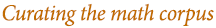So how big is the historical corpus of mathematics? There’ve probably been about 3 million mathematical papers published altogether—or about 100 million pages, growing at a rate of about 2 million pages per year. And in all of these papers, perhaps 5 million distinct theorems have been formally stated.

So what can be done with these? First, of course, there’s simple search and retrieval. Often the words in the papers will make for better search targets than the more notational material in the actual theorems. But with the kind of linguistic-understanding technology for math that we have in Wolfram|Alpha, it should not be too difficult to build what’s needed to do good statistical retrieval on the corpus of mathematical papers.

But can one go further? One might think about tagging the source documents to improve retrieval. But my guess is that most kinds of static tagging won’t be worth the trouble; just as one’s seen for the web in general, it’ll be much easier and better to make the search system more sophisticated and content-aware than to add tags document by document.

What would unquestionably be worthwhile, however, is to put the theorems into a genuine computable form: to actually take theorems from papers and rewrite them in a precise symbolic language.

Will it be possible to do this automatically? Eventually I suspect large parts of it will. Today we can take small fragments of theorems from papers and use the linguistic understanding system built for Wolfram|Alpha to turn them into pieces of Wolfram Language code. But it should gradually be possible to extend this to larger fragments—and eventually get to the point where it takes, at most, modest human effort to convert a typical theorem to precise symbolic form.

So let’s imagine we curate all the theorems from the literature of mathematics, and get them in computable form. What would we do then? We could certainly build a Wolfram|Alpha-like system that would be quite spectacular—and very useful in practice for doing lots of pure mathematics.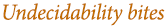But there will inevitably be some limitations—resulting in fact from features of mathematics itself. For example, it won’t necessarily be easy to tell what theorem might apply to what, or even what theorems might be equivalent. Ultimately these are classic theoretically undecidable problems—and I suspect that they will often actually be difficult in practical cases too. And at the very least, all of them involve the same kind of basic process as automated theorem proving.

And what this suggests is a kind of combination of the two basic approaches we’ve discussed—where in effect one takes the complete corpus of published mathematics, and views it as defining a giant 5-million-axiom formal system, and then follows the kind of automated theorem-enumeration procedure we discussed to find “interesting things to say”.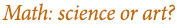So, OK, let’s say we build a wonderful system along these lines. Is it actually solving a core problem in doing pure mathematics, or is it missing the point?

I think it depends on what one sees the nature of the pure mathematical enterprise as being. Is it science, or is it art? If it’s science, then being able to make more theorems faster is surely good. But if it’s art, that’s really not the point. If doing pure mathematics is like creating a painting, automation is going to be largely counterproductive—because the core of the activity is in a sense a form of human expression.

This is not unrelated to the role of proof. To some mathematicians, what matters is just the theorem: knowing what’s true. The proof is essentially backup to ensure one isn’t making a mistake. But to other mathematicians, proof is a core part of the content of the mathematics. For them, it’s the story that brings mathematical concepts to light, and communicates them.

So what happens when we generate a proof automatically? I had an interesting example about 15 years ago, when I was working on A New Kind of Science, and ended up finding the simplest axiom system for Boolean algebra (just the single axiom ((p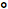q)r)(p((pr)p))==r, as it turned out). I used equational-logic automated theorem-proving (now built into FullSimplify) to prove the correctness of the axiom system. And I printed the proof that I generated in the book: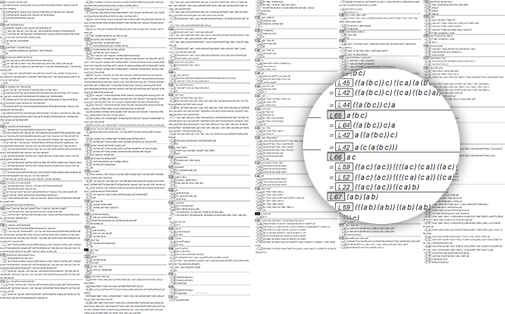It has 343 steps, and in ordinary-size type would be perhaps 40 pages long. And to me as a human, it’s completely incomprehensible. One might have thought it would help that the theorem prover broke the proof into 81 lemmas. But try as I might, I couldn’t really find a way to turn this automated proof into something I or other people could understand. It’s nice that the proof exists, but the actual proof itself doesn’t tell me anything.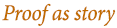And the problem, I think, is that there’s no “conceptual story” around the elements of the proof. Even if the lemmas are chosen “structurally” as good “waypoints” in the proof, there are no cognitive connections—and no history—around these lemmas. They’re just disembodied, and apparently disconnected, facts.

So how can we do better? If we generate lots of similar proofs, then maybe we’ll start seeing similar lemmas a lot, and through being familiar they will seem more meaningful and comprehensible. And there are probably some visualizations that could help us quickly get a good picture of the overall structure of the proof. And of course, if we manage to curate all known theorems in the mathematics literature, then we can potentially connect automatically generated lemmas to those theorems.

It’s not immediately clear how often that will possible. And indeed, in existing examples of computer-assisted proofs—like for the Four Color Theorem, the Kepler Conjecture, or the simplest universal Turing machine—my impression is that their often-computer-generated lemmas rarely correspond to known theorems from the literature.

But despite all this, I know at least one example showing that with enough effort, one can generate proofs that tell stories that people can understand: the step-by-step solutions system in Wolfram|Alpha Pro. Millions of times a day students and others compute things like integrals with Wolfram|Alpha—then ask to see the steps.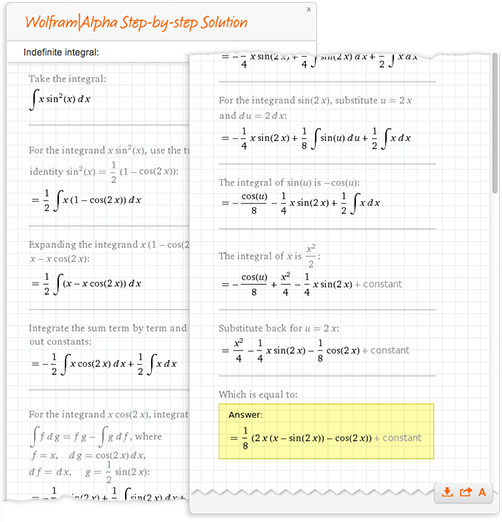It’s notable that actually computing the integral is much easier than figuring out good steps to show; in fact, it takes some fairly elaborate algorithms and heuristics to generate steps that successfully communicate to a human how the integral can be done. But the example of step-by-step in Wolfram|Alpha suggests that it’s at least conceivable that with enough effort, it would be possible to generate proofs that are readable as “stories”—perhaps even selected to be as short and simple as possible (“proofs from The Book”, as Erdős would say).

Of course, while these kinds of automated methods may eventually be good at communicating the details of something like a proof, they won’t realistically be able to communicate—or even identify—overarching ideas and motivations. Needless to say, present-day pure mathematics papers are often quite deficient in communicating these too. Because in an effort to ensure rigor and precision, many papers tend to be written in a very formal way that cannot successfully represent the underlying ideas and motivations in the mind of the author—with the result that some of the most important ideas in mathematics are transmitted through an essentially oral tradition.

It would certainly help the progress of pure mathematics if there were better ways to communicate its content. And perhaps having a precise symbolic language for pure mathematics would make it easier to express concretely some of those important points that are currently left unwritten. But one thing is for sure: having such a language would make it possible to take a theorem from anywhere, and—like with a typical Wolfram Language code fragment—immediately be able to plug it in anywhere else, and use it.

But back to the question of whether automation in pure mathematics can ultimately make sense. I consider it fairly clear that a Wolfram|Alpha-like “pure math assistant” would be useful to human mathematicians. I also consider it fairly clear that having a good, precise, symbolic language—a kind of Mathematica Pura that’s a well-designed follow-on to standard mathematical notation—would be immensely helpful in formulating, checking and communicating math.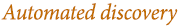But what about a computer just “going off and doing math by itself”? Obviously the computer can enumerate theorems, and even use heuristics to select ones that might be considered interesting to human mathematicians. And if we curate the literature of mathematics, we can do extensive “empirical metamathematics” and start trying to recognize theorems with particular characteristics, perhaps by applying graph-theoretic criteria on the network of theorems to see what counts as “surprising” or a “powerful” theorem. There’s also nothing particularly difficult—like in WolframTones—about having the computer apply aesthetic criteria deduced from studying human choices.

But I think the real question is whether the computer can build up new conceptual frameworks and structures—in effect new mathematical theories. Certainly some theorems found by enumeration will be surprising and indicative of something fundamentally new. And it will surely be impressive when a computer can take a large collection of theorems—whether generated or from the literature—and discover correlations among them that indicate some new unifying principle. But I would expect that in time the computer will be able not only to identify new structures, but also name them, and start building stories about them. Of course, it is for humans to decide whether they care about where the computer is going, but the basic character of what it does will, I suspect, be largely indistinguishable from many forms of human pure mathematics.

All of this is still fairly far in the future, but there’s already a great way to discover math-like things today—that’s not practiced nearly as much as it should be: experimental mathematics. The term has slightly different meanings to different people. For me it’s about going out and studying what mathematical systems do by running experiments on them. And so, for example, if we want to find out about some class of cellular automata, or nonlinear PDEs, or number sequences, or whatever, we just enumerate possible cases and then run them and see what they do.

There’s a lot to discover like this. And certainly it’s a rich way to generate observations and hypotheses that can be explored using the traditional methodologies of pure mathematics. But the real thrust of what can be done does not fit into what pure mathematicians typically think of as math. It’s about exploring the “flora and fauna”—and principles—of the universe of possible systems, not about building up math-like structures that can be studied and explained using theorems and proofs. Which is why—to quote the title of my book—I think one should best consider this a new kind of science, rather than something connected to existing mathematics.

In discussing experimental mathematics and A New Kind of Science, it’s worth mentioning that in some sense it’s surprising that pure mathematics is doable at all—because if one just starts asking absolutely arbitrary questions about mathematical systems, many of them will end up being undecidable.

This is particularly obvious when one’s out in the computational universe of possible programs, but it’s also true for programs that represent typical mathematical systems. So why isn’t undecidability more of a problem for typical pure mathematics? The answer is that pure mathematics implicitly tends to select what it studies so as to avoid undecidability. In a sense this seems to be a reflection of history: pure mathematics follows what it has historically been successful in doing, and in that way ends up navigating around undecidability—and producing the millions of theorems that make up the corpus of existing pure mathematics.

OK, so those are some issues and directions. But where are we at in practice in bringing computational knowledge to pure mathematics?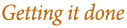There’s certainly a long history of related efforts. The works of Peano and Whitehead and Russell from a century ago. Hilbert’s program. The development of set theory and category theory. And by the 1960s, the first computer systems—such as Automath—for representing proof structures. Then from the 1970s, systems like Mizar that attempted to provide practical computer frameworks for presenting proofs. And in recent times, increasingly popular “proof assistants” based on systems like Coq and HOL.

One feature of essentially all these efforts is that they were conceived as defining a kind of “low-level language” for mathematics. Like most of today’s computer languages, they include a modest number of primitives, then imagine that essentially any actual content must be built externally, by individual users or in libraries.

But the new idea in the Wolfram Language is to have a knowledge-based language, in which as much actual knowledge as possible is carefully designed into the language itself. And I think that just like in general computing, the idea of a knowledge-based language is going to be crucial for injecting computation into pure mathematics in the most effective and broadly useful way.

So what’s involved in creating our Mathematica Pura—an extension to the Wolfram Language that builds in the actual structure and content of pure math? At the lowest level, the Wolfram Language deals with arbitrary symbolic expressions, which can represent absolutely anything. But then the language uses these expressions for many specific purposes. For example, it can use a symbol x to represent an algebraic variable. And given this, it has many functions for handling symbolic expressions—interpreted as mathematical or algebraic expressions—and doing various forms of math with them.

The emphasis of the math in Mathematica and the Wolfram Language today is on practical, calculational, math. And by now it certainly covers essentially all the math that has survived from the 19th century and before. But what about more recent math? Historically, math itself went through a transition about a century ago. Just around the time modernism swept through areas like the arts, math had its own version: consider systems that emerged purely from its own formalism, without regard for obvious connection to the outside world.

And this is the kind of math—through developments like Bourbaki and beyond—that came to dominate pure mathematics in the 20th century. And inevitably, a lot of this math is about defining abstract structures to study. In simple cases, it seems like one might represent these structures using some hierarchy of types. But the types need to be parametrized, and quite quickly one ends up with a whole algebra or calculus of types—and it’s just as well that in the Wolfram Language one can use general symbolic expressions, with arbitrary heads, rather than just simple type descriptions.

As I mentioned early in this blog post, it’s going to take all sorts of new built-in functions to capture the frameworks needed to represent modern pure mathematics—together with lots of entity-like objects. And it’ll certainly take years of careful design to make a broad system for pure mathematics that’s really clean and usable. But there’s nothing fundamentally difficult about having symbolic constructs that represent differentiability or moduli spaces or whatever. It’s just language design, like designing ways to represent 3D images or remote computation processes or unique external entity references.

So what about curating theorems from the literature? Through Wolfram|Alpha and the Wolfram Language, not to mention for example the Wolfram Functions Site and the Wolfram Connected Devices Project, we’ve now had plenty of experience at the process of curation, and in making potentially complex things computable.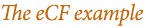But to get a concrete sense of what’s involved in curating mathematical theorems, we did a pilot project over the last couple of years through the Wolfram Foundation, supported by the Sloan Foundation. For this project we picked a very specific and well-defined area of mathematics: research on continued fractions. Continued fractions have been studied continually since antiquity, but were at their most popular between about 1780 and 1910. In all there are around 7000 books and papers about them, running to about 150,000 pages.

We chose about 2000 documents, then set about extracting theorems and other mathematical information from them. The result was about 600 theorems, 1500 basic formulas, and about 10,000 derived formulas. The formulas were directly in computable form—and were in effect immediately able to join the 300,000+ on the Wolfram Functions Site, that are all now included in Wolfram|Alpha. But with the theorems, our first step was just to treat them as entities themselves, with properties such as where they were first published, who discovered them, etc. And even at this level, we were able to insert some nice functionality into Wolfram|Alpha.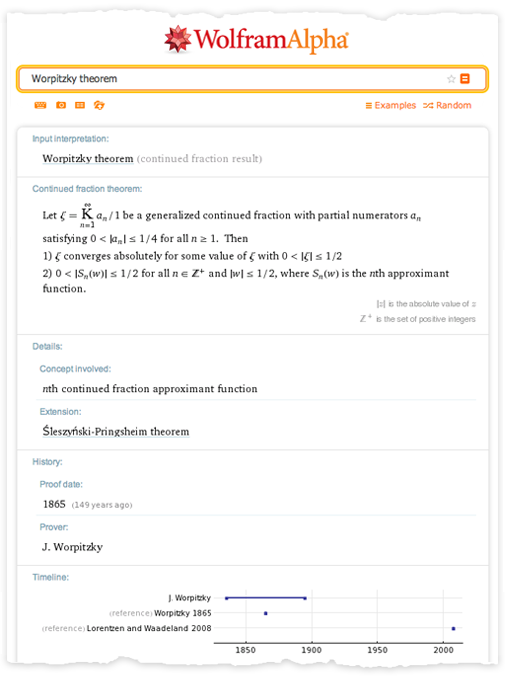But we also started trying to actually encode the content of the theorems in computable form. It took introducing some new constructs like LebesgueMeasure, ConvergenceSet and LyapunovExponent. But there was no fundamental problem in creating precise symbolic representations of the theorems. And just from these representations, it became possible to do computations like this in Wolfram|Alpha:

An interesting feature of the continued fraction project (dubbed “eCF”) was how the process of curation actually led to the discovery of some new mathematics. For having done curation on 50+ papers about the Rogers–Ramanujan continued fraction, it became clear that there were missing cases that could now be computed. And the result was the filling of a gap left by Ramanujan for 100 years.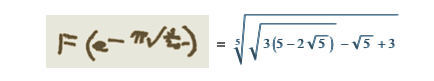There’s always a tradeoff between curating knowledge and creating it afresh. And so, for example, in the Wolfram Functions Site, there was a core of relations between functions that came from reference books and the literature. But it was vastly more efficient to generate other relations than to scour the literature to find them.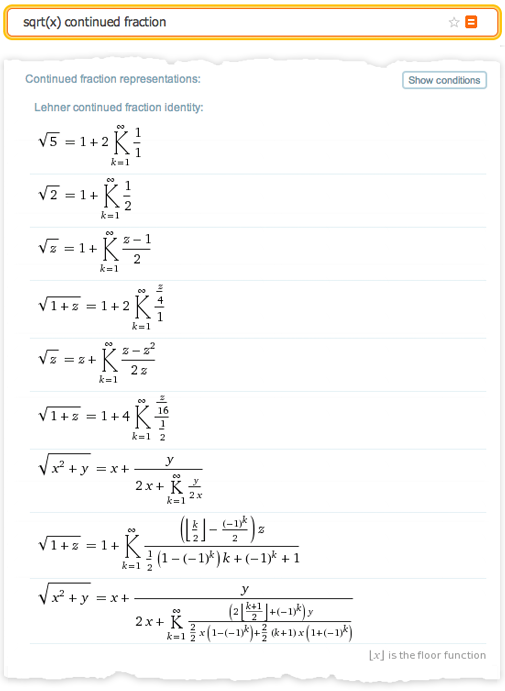But if the goal is curation, then what would it take to curate the complete literature of mathematics? In the eCF project, it took about 3 hours of mathematician time to encode each theorem in computable form. But all this work was done by hand, and in a larger-scale project, I am certain that an increasing fraction of it could be done automatically, not least using extensions of our Wolfram|Alpha natural language understanding system.

Of course, there are all sorts of practical issues. Newer papers are predominantly in TeX, so it’s not too difficult to pull out theorems with all their mathematical notation. But older papers need to be scanned, which requires math OCR, which has yet to be properly developed.

Then there are issues like whether theorems stated in papers are actually valid. And even whether theorems that were considered valid, say, 100 years ago are still considered valid today. For example, for continued fractions, there are lots of pre-1950 theorems that were successfully proved in their time, but which ignore branch cuts, and so wouldn’t be considered correct today.

And in the end of course it requires lots of actual, skilled mathematicians to guide the curation process, and to encode theorems. But in a sense this kind of mobilization of mathematicians is not completely unfamiliar; it’s something like what was needed when Zentralblatt was started in 1931, or Mathematical Reviews in 1941. (As a curious footnote, the founding editor of both these publications was Otto Neugebauer, who worked just down the hall from me at the Institute for Advanced Study in the early 1980s, but who I had no idea was involved in anything other than decoding Babylonian mathematics until I was doing research for this blog post.)

When it comes to actually constructing a system for encoding pure mathematics, there’s an interesting example: Theorema, started by Bruno Buchberger in 1995, and recently updated to version 2. Theorema is written in the Wolfram Language, and provides both a document-based environment for representing mathematical statements and proofs, and actual computation capabilities for automated theorem proving and so on.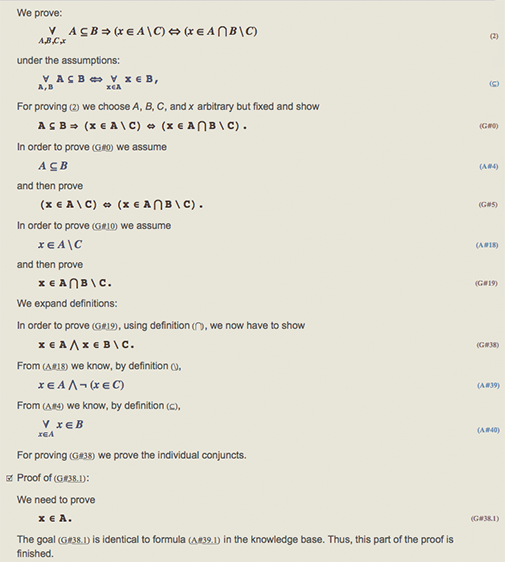No doubt it’ll be an element of what’s ultimately built. But the whole project is necessarily quite large—perhaps the world’s first example of “big math”. So can the project get done in the world today? A crucial part is that we now have the technical capability to design the language and build the infrastructure that’s needed. But beyond that, the project also needs a strong commitment from the world’s mathematics community—as well as lots of contributions from individual mathematicians from every possible field. And realistically it’s not a project that can be justified on commercial grounds—so the likely \$100+ million that it will need will have to come from non-commercial sources.

But it’s a great and important project—that promises to be pivotal for pure mathematics. In almost every field there are golden ages when dramatic progress is made. And more often than not, such golden ages are initiated by new methodology and the arrival of new technology. And this is exactly what I think will happen in pure mathematics. If we can mobilize the effort to curate known mathematics and build the system to use and generate computational knowledge around it, then we will not only succeed in preserving and spreading the great heritage of pure mathematics, but we will also thrust pure mathematics into a period of dramatic growth.

Large projects like this rely on strong leadership. And I stand ready to do my part, and to contribute the core technology that is needed. Now to move this forward, what it takes is commitment from the worldwide mathematics community. We have the opportunity to make the second decade of the 21st century really count in the multi-millennium history of pure mathematics. Let’s actually make it happen!

To comment, please visit the original post at the Stephen Wolfram Blog »